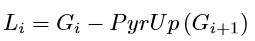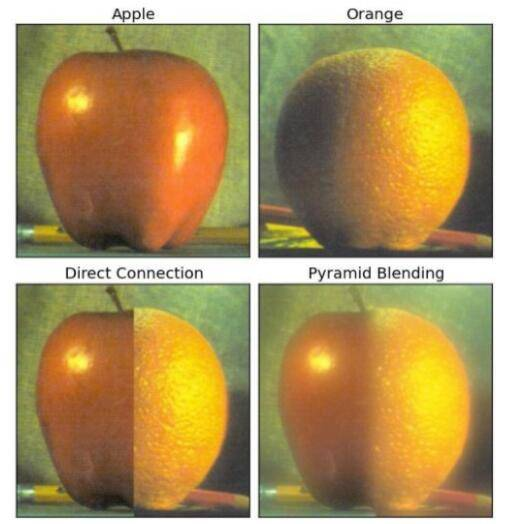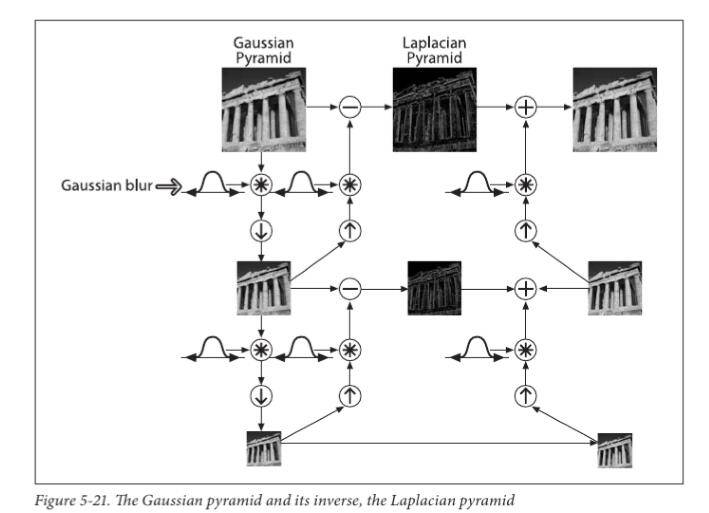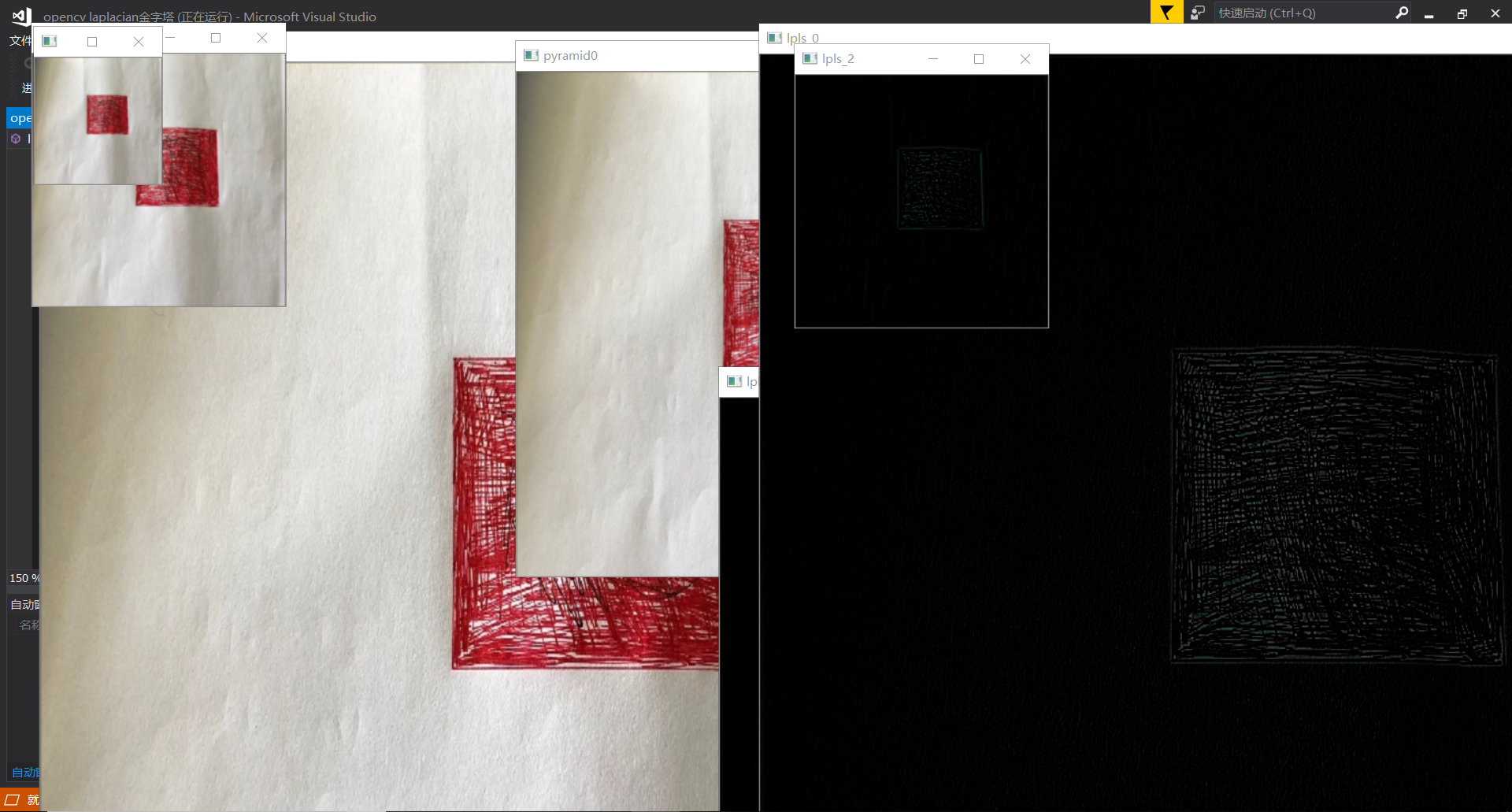129
0
2021年1月3日 09时33分

## 1.1高斯金字塔

cv2.pyrDown从一个高分辨率大尺寸的图像向上构建一个金字塔（尺寸变小，分辨率降低）

cv2.U拍从一个小尺寸的图像向上构建一个金字塔（尺寸变大，总体分辨率不变，但因为总体变大了，所以看上去分辨率还是降低的。）

import numpy as np
import cv2

lower_reso = cv2.pyrDown(img)

while(1):
cv2.imshow('img',img)
cv2.imshow('lower_reso',lower_reso)
if cv2.waitKey() == ord('q'):
break
cv2.destroyAllWindows()
cv2.pyrUp从一个低分辨率小尺寸的图像向上构建一个金字塔（尺寸变大，但分辨率不会增加）

import numpy as np
import cv2

lower_reso = cv2.pyrDown(img)
higher_reso2 = cv2.pyrUp(img)

while(1):
cv2.imshow('img',img)
cv2.imshow('lower_reso',lower_reso)
cv2.imshow('higher_reso2',higher_reso2)
if cv2.waitKey() == ord('q'):
break
cv2.destroyAllWindows()

## 1.2.拉普拉斯金字塔## 2.使用金字塔进行图像融合·读入两幅图

·构建各自的高斯金字塔（6层）

·根据高斯金字塔计算拉普拉斯金字塔

·在拉普拉斯的每一层进行图像融合

·根据融合后的图像金字塔重建原始图像。

·如何重建原始图像过程import cv2
#高斯金字塔
def pyramid_demo(image):
level = 3      #设置金字塔的层数为3
temp = image.copy()  #拷贝图像
pyramid_images = []  #建立一个空列表
for i in range(level):
dst = cv2.pyrDown(temp)   #先对图像进行高斯平滑，然后再进行降采样（将图像尺寸行和列方向缩减一半）
pyramid_images.append(dst)  #在列表末尾添加新的对象
cv2.imshow("pyramid"+str(i), dst)
temp = dst.copy()
return pyramid_images
#拉普拉斯金字塔
def lapalian_demo(image):
pyramid_images = pyramid_demo(image)    #做拉普拉斯金字塔必须用到高斯金字塔的结果
level = len(pyramid_images)
for i in range(level-1, -1, -1):
if (i-1) < 0:
expand = cv2.pyrUp(pyramid_images[i], dstsize = image.shape[:2])
lpls = cv2.subtract(image, expand)
cv2.imshow("lapalian_down_"+str(i), lpls)
else:
expand = cv.pyrUp(pyramid_images[i], dstsize = pyramid_images[i-1].shape[:2])
lpls = cv.subtract(pyramid_images[i-1], expand)
cv2.imshow("lapalian_down_"+str(i), lpls)
cv2.namedWindow('input_image', cv.WINDOW_AUTOSIZE) #设置为WINDOW_NORMAL可以任意缩放
cv2.imshow('input_image', src)
lapalian_demo(src)
cv2.waitKey(0)
cv2.destroyAllWindows()## 总结：

（本系列每周不定期更新，谢谢大家的支持！）